# How to Calculate and Solve for Unit Hydrograph | Hydrology II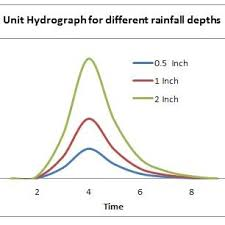The image above represents unit hydrograph.

To compute for unit hydrograph, two essential parameters are needed and these parameters are Direct run off (DRO) and Effective depth (ED).

The formula for calculating unit hydrograph:

UHG = DRO / ED

Where:

UHG = Unit Hydrograph
DRO = Direct Run Off
ED = Effective Depth

Let’s solve an example;
Find the unit hydrograph when the direct run off is 11 and the effective depth is 13.

This implies that:

DRO = Direct Run Off = 11
ED = Effective Depth = 13

UHG = DRO / ED
UHG = 11 / 13
UHG = 0.846

Therefore, the unit hydrograph is 0.846.

Calculating the Direct Run Off when the Unit Hydrograph and the Effective Depth is Given.

DRO = UHG x ED

Where;

DRO = Direct Run Off
UHG = Unit Hydrograph
ED = Effective Depth

Let’s solve an example;
Find the direct run off when the unit hydrograph is 21 and the effective depth is 10.

This implies that;

UHG = Unit Hydrograph = 21
ED = Effective Depth = 10

DRO = UHG x ED
DRO = 21 x 10
DRO = 210

Therefore, the direct run off is 210.

Calculating the Effective Depth when the Unit Hydrograph and the Direct Run Off is Given.

ED = DRO / UHG

Where;

ED = Effective Depth
DRO = Direct Run Off
UHG = Unit Hydrograph

Let’s solve an example;
Find the Effective Depth when the Direct Run Off is 250 and the unit hydrograph is 50.

This implies that;

DRO = Direct Run Off = 250
UHG = Unit Hydrograph = 50

ED = DRO / UHG
ED = 250 / 50
ED = 5

Therefore, the effective depth is 5.

Nickzom Calculator – The Calculator Encyclopedia is capable of calculating the unit hydrograph.

To get the answer and workings of the unit hydrograph using the Nickzom Calculator – The Calculator Encyclopedia. First, you need to obtain the app.

You can get this app via any of these means:

To get access to the professional version via web, you need to register and subscribe for NGN 2,000 per annum to have utter access to all functionalities.
You can also try the demo version via https://www.nickzom.org/calculator

Apple (Paid) – https://itunes.apple.com/us/app/nickzom-calculator/id1331162702?mt=8
Once, you have obtained the calculator encyclopedia app, proceed to the Calculator Map, then click on Agricultural under Engineering.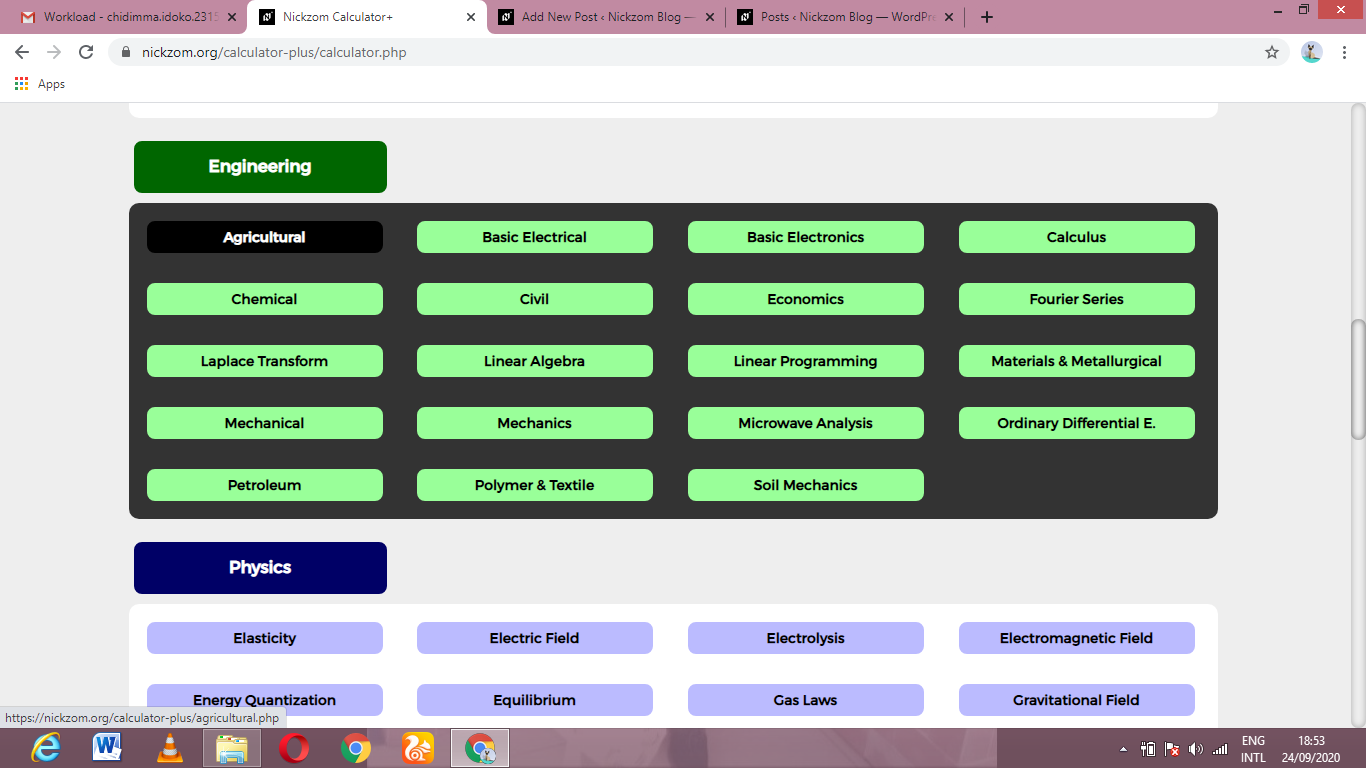Now, Click on Hydrology II under Agricultural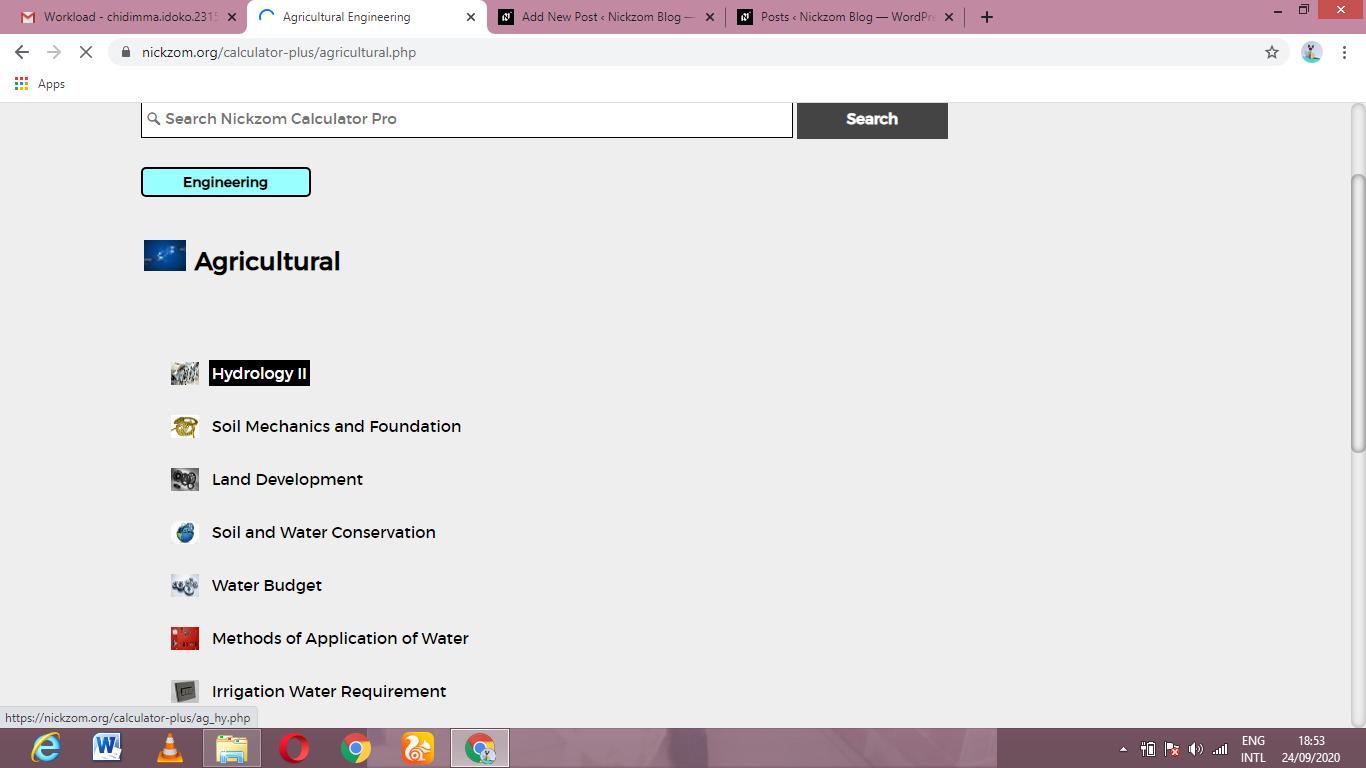Now, Click on Unit Hydrograph under Hydrology II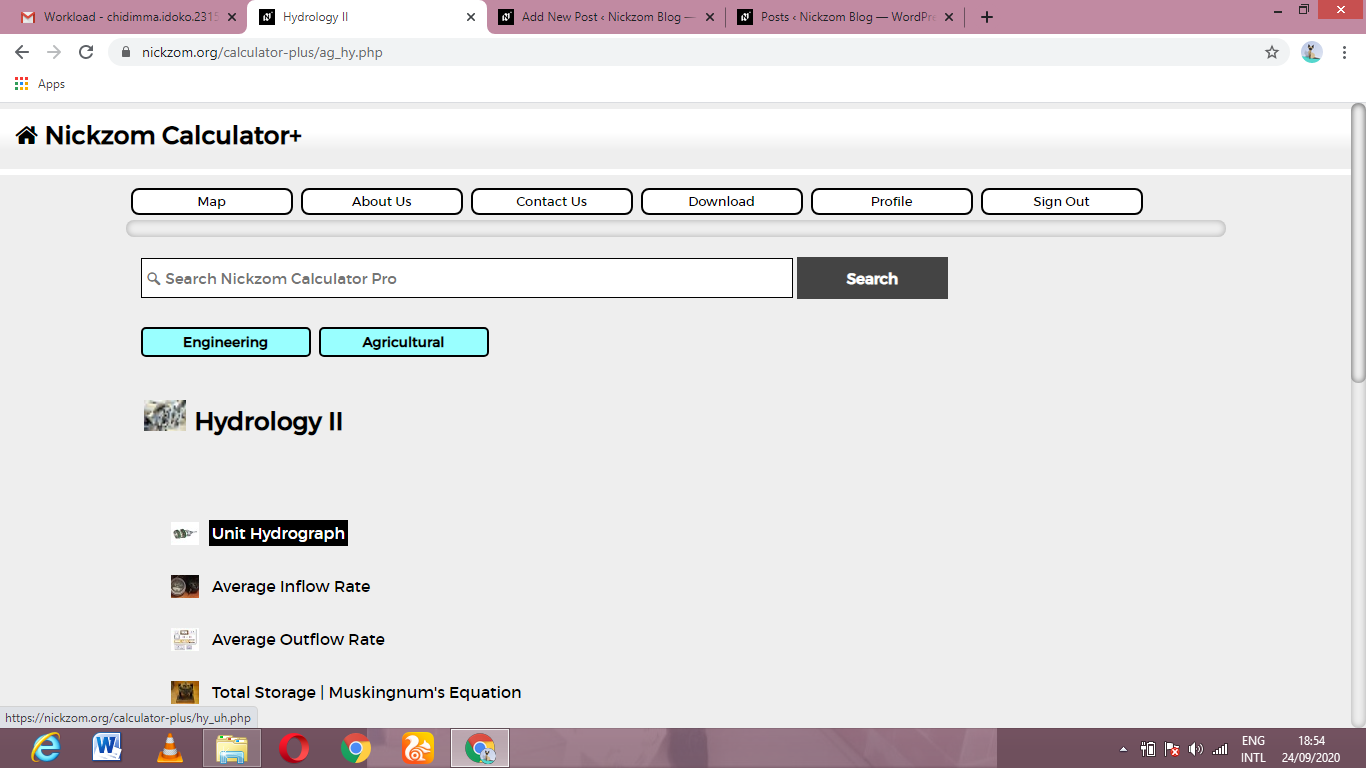The screenshot below displays the page or activity to enter your values, to get the answer for the unit hydrograph according to the respective parameters which is the Direct run off (DRO) and Effective depth (ED).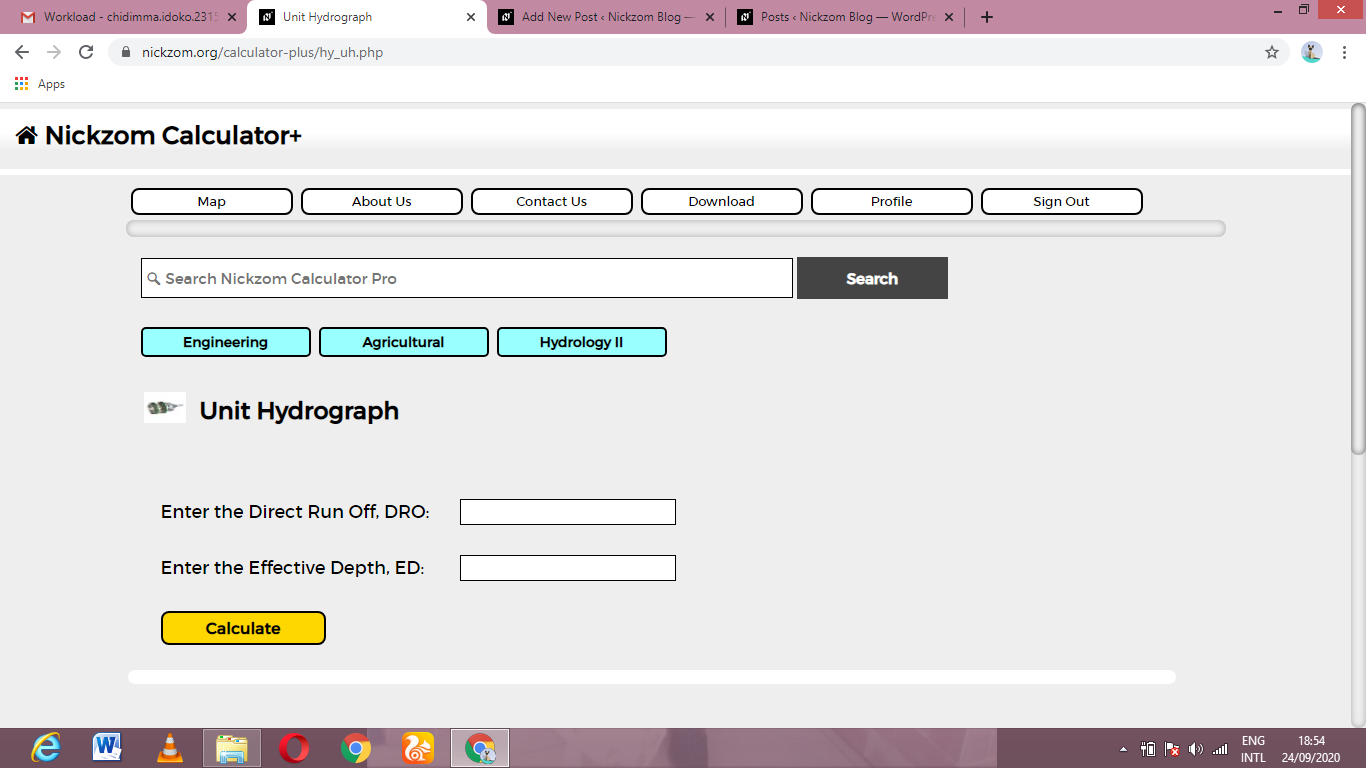Now, enter the values appropriately and accordingly for the parameters as required by the Direct run off (DRO) is 11 and Effective depth (ED) is 13.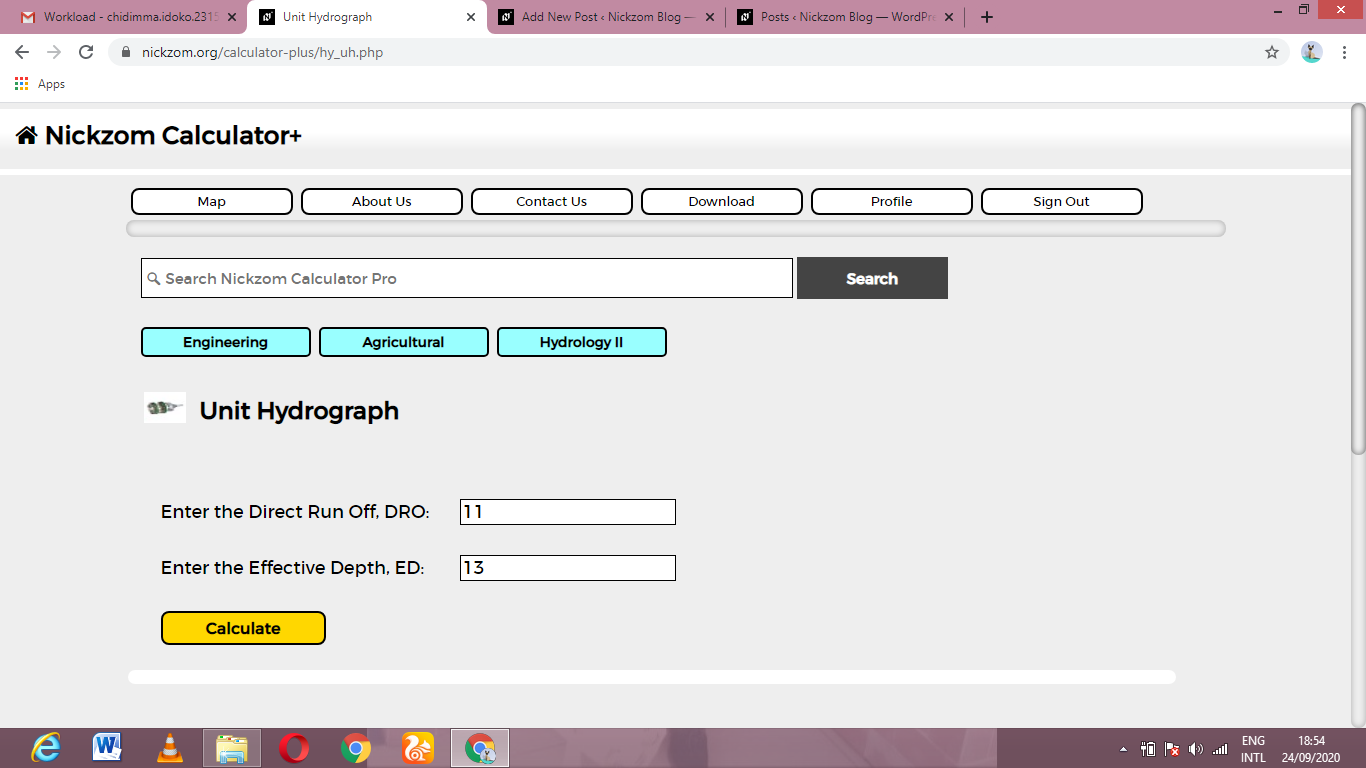Finally, Click on Calculate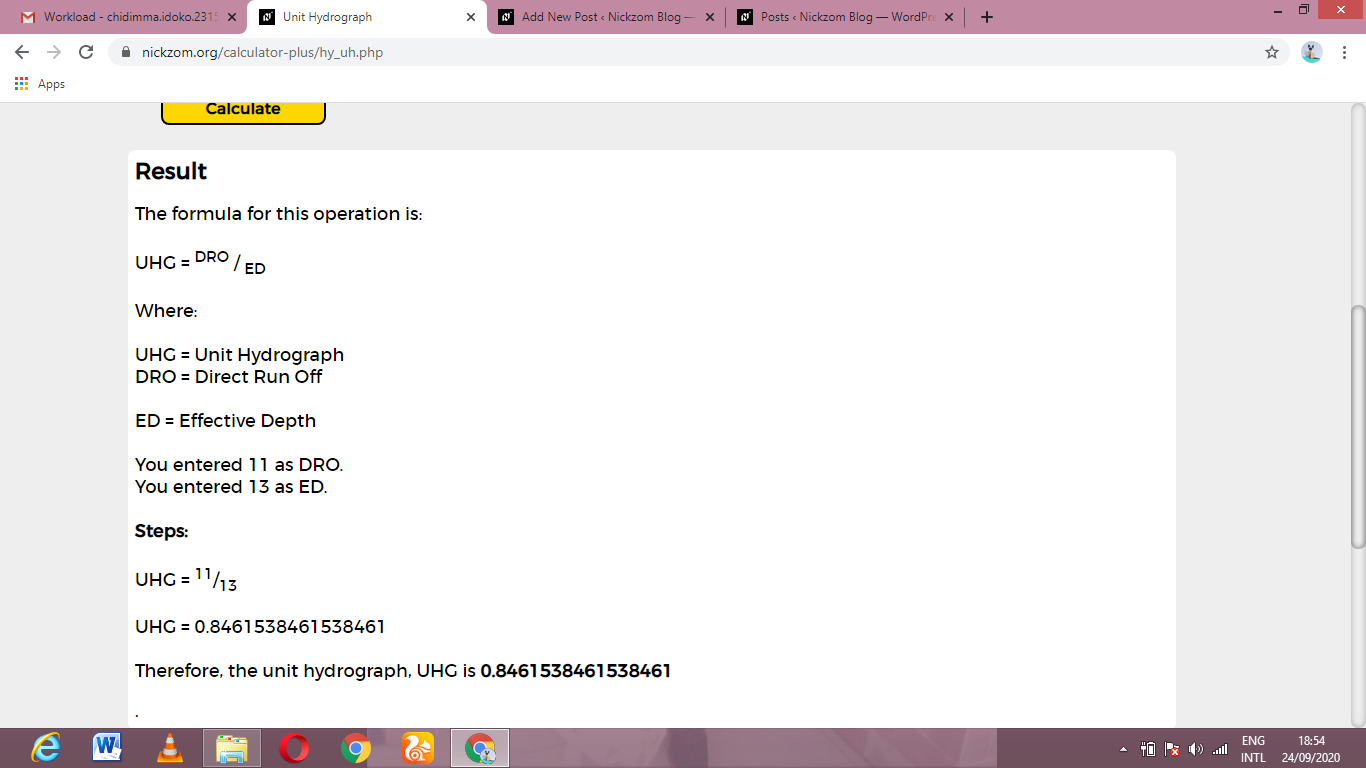As you can see from the screenshot above, Nickzom Calculator– The Calculator Encyclopedia solves for the unit hydrograph and presents the formula, workings and steps too.